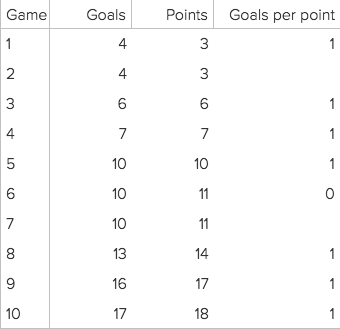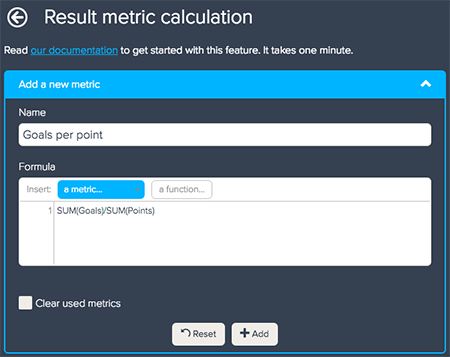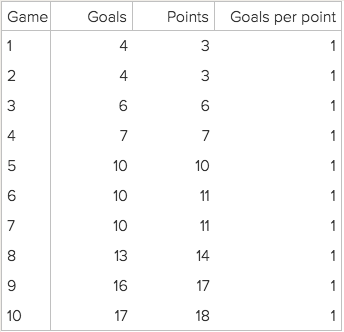# Performing calculations on manipulated results

Follow

The result metric calculation enables you to create calculations based on already manipulated metrics.

If you want to calculate a rolling conversion rate or 'per X' calculation between two metrics, using a calculated metric might not provide you with the correct results. For example, if you are creating a report to view the goals per point a team is managing, using calculated metrics will not base calculations on the already manipulated metrics.

Note: The examples in this article use metrics with running totals already applied. To calculate a rolling conversion rate, you must first create running totals. For information on creating running totals, see Creating result path calculations.

Below is an example of a report using calculated metrics.To accurately view the goals per point, you would need to set up a result metric calculation.

To create a result metric calculation

1. Open the Result manipulation menu, then select Result metric calculation
2. Click Add a new metric.
3. Enter the name of your metric.
4. Type in a formula using the full names of the metrics involved and simple mathematical operators. The example below demonstrates the goals per point calculation.5. Check the Clear used metrics box to hide all of the other metric results on your query, except for the result metric calculation. The metrics are still used in the result metric calculation, but the results will not appear in your chart.
6. Click the Add button to add the new result metric calculation.